In this video, we are going to look at how to evaluate quadratic functions. Quadratic functions are written in the form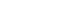$y=ax^2+bx+c$ or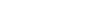$f(x)=ax^2+bx+c$. After you finish this lesson, view all of our Algebra 1 lessons and practice problems.

For example:
When given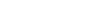$y=3x^2+2x-5$ at x=2, we substitute 2 in for where we see x.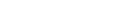$y=3(2)^2+2(2)-5$
Remember to always plug in values in parenthesis (to avoid confusion with signs). Then, this can be simplified to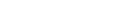$y=3(4)+2(2)-5$
and even further to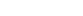$y=12+4-5$
to get a final answer of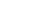$y=11$

## Example of Evaluating Quadratic Functions

### Example 1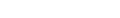$y=x^2-7x-13$ where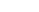$x = 6$

So let’s substitute.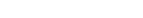$y=(6)^2-7(6)-13$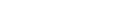$y=36-42-13$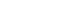$y=-19$

### Example 2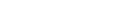$f(x) = 4b^2+6b-4$ ; find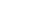$f(5)$
So let’s substitute.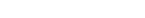$f(5)=4(5)^2+6(5)-4$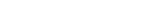$f(5)=4(25)+6(5)-4$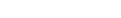$f(5)=100+35-4$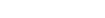$f(5)=135-4$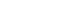$f(5)=131$

## Video-Lesson Transcript

Let’s go over how to evaluate quadratic functions.$y = ax^2 + bx + c$

The most important thing to note here is the presence of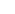$x^2$. This is the highest exponent.

Or this form:$f(x) = ax^2 + bx + c$$y = 3x^2 + 2x - 5$ where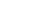$x = 2$

So let’s substitute.$y = 3 (2)^2 + 2 (2) - 5$$y = 3 (4) + 2 (2) - 5$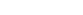$y = 12 + 4 - 5$$y = 11$Let’s look at another example in a different form.$f(x) = 2x^2 - 4x + 7$,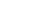$f(-3)$

Now, let’s substitute.$f(x) = 2x^2 - 4x + 7$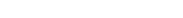$f(-3) = 2 (-3)^2 - 4 (-3) + 7$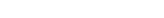$f(-3) = 2 (9) - 4 (-3) + 7$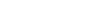$f(-3) = 18 + 12 + 7$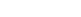$f(-3) = 37$